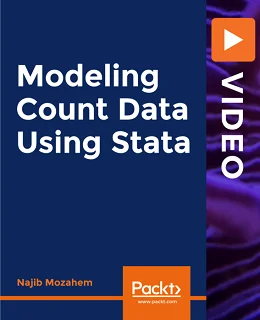# Modeling Count Data using Stata [Video]### Modeling Count Data using Stata [Video]

##### English | MP4 | AVC 1920×1080 | AAC 44KHz 2ch | 4h 40m | 1.25 GB eLearning | Skill level: All Levels

Modeling Count Data using Stata [Video]: Learn Poisson and negative binomial regression techniques

The course is divided into two parts. In the first part, you’ll be introduced to the theory behind count models in an intuitive way while keeping the math at a minimum. The course starts with an overview of count tables, where you’ll learn how to calculate the incidence rate ratio. You’ll get to grips with Poisson regression and understand how to work with continuous, binary, and categorical variables. As you advance, you’ll explore the concept of overdispersion and how to address this issue using negative binomial models. The course also covers other count models such as truncated models and zero-inflated models.

• Calculate incidence rate ratios
• Identify when to use count models
• Apply count models using Stata
• Predict the expected number of outcomes
• Compare different models
• Understand what count models are

In the second part of the course, you’ll be able to apply what you have learned using Stata. You’ll be taken through a large project where you’ll fit the Poisson, negative binomial, and zero-inflated models. Additionally, you’ll discover the tools used to compare these models.